# 13.10: Multinomial Distributions

•• Peter Woolf et al.
• Assistant Professor (Chemical Engineering) at University of Michigan

## Introduction

Statistical events generate two types of outcomes: continuous or discrete. Continuous outcomes can take an infinite number of values; for instance, a person's height can take any real number, so there are infinite possibilities. Typical events generating continuous outcomes may follow a normal, exponential, or geometric distribution. Discrete outcomes can only on take prescribed values; for instance, a dice roll can only generate an integer between 1 to 6. Discrete outcomes are typically distributed either binomially or multinomially. It is with multinomial distribution that this article is concerned.

## Multinomial Distributions: Mathematical Representation

Multinomial distributions specifically deal with events that have multiple discrete outcomes. The Binomial distribution is a specific subset of multinomial distributions in which there are only two possible outcomes to an event.

Multinomial distributions are not limited to events only having discrete outcomes. It is possible to categorize outcomes with continuous distributions to different degrees (high, medium, low). For instance, the water level - a continuous entity - in a storage tank can be made discrete by categorizing them into either "desirable" or "not desirable." Multinomial distributions, therefore, have expansive applications in process control.

### Probability Density Function

One way of describing the probability of an outcome occurring in a trial is the probability density function. The probability density function (PDF) mathematically represents the probability of having a specified outcome. The probability density function is a useful way to find the probability of simultaneous occurrence of specific results (i.e. having $$n_1 = 1$$, $$n_2 = 1$$, and $$n_3 = 1$$ with 3 trials as opposed to other outcomes, such as $$n_1 = 3$$, $$n_2 = 0$$, $$n_3 = 0$$ or $$n_1 = 0$$, $$n_2 = 1$$, $$n_3 = 2$$).

$P\left(n_{1}, n_{2}, \ldots, n_{k}\right)=\left(n_{1} ! n_{2} ! \ldots n_{k} !\right) \prod_{i=1}^{N !} p_{i}^{n_{i}}=\left(n_{1} ! n_{2} ! \ldots n_{l \ell} !\right) *\left(p_{1}^{n_{1}} p_{2}^{n_{2}} \ldots p_{k}^{n_{k}}\right)$

where

• $$N$$ is the number of trials
• $$k$$ is the number of possible outcomes
• $$n_i$$ is the number of occurrences of outcome $$i$$
• $$p_i$$ is the probability of observing outcome $$i$$

We know that the the sum of the probabilities of all possible outcomes that can occur in a trial must be unity (since one outcome must occur). The probability density function yields this result for both continuous and discrete outcomes. However, it is important to note that to get this result for continuous outcomes, one must take the integral of the probability density function over all possible outcomes. To get this unity result for discrete outcomes, one must sum the probabilities of each outcome (similar to taking Riemann sums).

### Cumulative Distribution Function

While the probability density function calculates the probability of a single outcome, the cumulative distribution function (CDF) is a useful way to find the probability that an outcome lies within a given range of values.where

• N is the number of trials
• k is the number of possible outcomes
• ni is the number of occurrences of outcome i
• pi is the probability of seeing outcome i
• ci is the maximum number of occurrences of outcome i

### Visualizing Probability Density Function with Mathematica

Before using the functions for multinomial probability distributions, a special package must be loaded using the following command (depending on the version of Mathematica):

<< Needs["MultivariateStatistics"] (Mathematica 6.0)

<< StatisticsMultiDiscreteDistributions (Mathematica 5.2)

Note that the  (tilde) is the key next to 1 and not the single quote character '.

Table of pertinent Mathematica commands:

Command

Description

PDF[multinomial,x]

probability density function at x, where x is scalar-, vector-, or matrix-valued depending on distribution

CDF[multinomial,x]

cumulative distribution function at x

Mean[multinomial]

mean

Variance[multinomial]

variance

StandardDeviation[multinomial]

standard deviation

To plot the multinomial distribution probability density function (PDF) in Mathematica, follow three simple steps:

Defining the Multinomial Distribution

multinomial = MultinomialDistribution[n,{p1,p2,...pk}] where k is the number of possible outcomes, n is the number of outcomes, and p1 to pk are the probabilities of that outcome occurring. n and p1 to pk are usually given as numbers but can be given as symbols as long as they are defined before the command.

Defining the PDF of the Multinomial Distribution

pdf=PDF[multinomial, {x1,x2,...,xk}]; The x here simply refers to the variable so this command can be typed as is, and leave the x as a variable not a number.

Plotting the PDF

Plot3D[pdf, {x1, 0, 6}, {x2, 0, 5}, AxesLabel -> {x1, x2, probability}] the 0,6 and 0,5 are the ranges of x1 and x2 on the plot respectively, and the AxesLabel argument is to help us see which is which on the plot created. This command can also be typed as is, by leaving all the x's as variables.

• Note that this will only work if you have 2 variables. If there are more variables, constraints can be set so that it can be plotted. For 3 variables, set the third variable x3 as n-x1-x2. See the attached Mathematica notebook for more information.

Media:Multinomial.nb

### Other Characteristics

Statistics have historically been useful in descriptive and inferential analysis of data. Likewise, multinomial distribution is also applicable to the aforementioned areas: descriptive statistics, inferential statistics, and six-sigma. Several key variables are used in these applications:

The expected value below describes the mean of the data. Discrete random variables can take on a range of values; the mean of the data describes the location of the data within this range.

$\mathrm{E}\left(X_{i}\right)=n p_{i}$

The data's standard deviation describes the variance of the data. The standard deviation of the data describes the spread of the data with respect to the center value (the mean of the data).

$\operatorname{var}\left(X_{i}\right)=n p_{i}\left(1-p_{i}\right)$

Using the above parameters, it is possible to find the probability of data lying within n standard deviations of the mean. By setting n equal to 6, six sigma quality control can be implemented on the event and outcomes in question.

### Derivation of Binomial Distribution

As was stated above, the binomial distribution is simply a special case of the multinomial distribution. Using the multinomial distribution, the probability of obtaining two events n1 and n2 with respective probabilities $$p_1$$ and $$p_2$$ from $$N$$ total is given by:

$P\left(n_{1}, n_{2}\right)=\frac{N !}{n_{1} ! n_{2} !}\left(p_{1}^{n_{1}} p_{2}^{n_{2}}\right)$

If we label the event of interest, say n1 in this case, as "k," then, since only two outcomes are possible, n2 must equal N-k. Finally, If we label the probability of obtaining k as simply "p," then the probability of obtaining event n2 (p2) must be 1-p, because again only two outcomes are possible. With these subsitutions, the above equation simplifies to

$P(k, N, p)=\frac{N !}{k !(N-k) !} p^{k}(1-p)^{N-k}$

Which is the familiar binomial distribution, where k is the number of events of interest, N is the total number of events, and p is the probability of attaining the event of interest.

## Applications of Multinomial Distributions

As mentioned before, multinomial distributions are a generalized version of binomial distributions. In chemical engineering applications, multinomial distributions are relevant to situations where there are more than two possible outcomes (temperature = {high, med, low}). Multinomial systems are a useful analysis tool when a “success-failure” description is insufficient to understand the system. A continuous form of the multinomial distribution is the Dirichlet distribution.

Using Bayes' Rule is one of the major applications of multinomial distributions. For example, Bayes' Rule can be used to predict the pressure of a system given the temperature and statistical data for the system. Bayes' Rule can be used to determine the probability of an event or outcome as mentioned above. Additional details on Bayes' Rule can be found at Bayes' Rule, conditional probability, independence.

### Bayes' Rule Example

In case you’ve forgotten how to use Bayes’ Rule, here is an example that shows how to use it to solve a problem with a multinomial outcome which results from combining dual possibilities (such as desired flow vs. undesired flow and configuration A vs. configuration B).

You are given a flow apparatus with two possible specific valve configurations, A and B. For specific valve configuration A, desired flow rates are achieved 98.5% of the time. And for specific valve configuration B, the desired flow rates are achieved 89.3% of the time. An operator might use the configuration from A 79% of the time when desired flow rates are achieved. Can you determine the probability that a randomly chosen configuration is the same as the one in specific valve configuration B and produces undesirable flow rates?

Worked Out Solution

We'll be using the following symbols:

FU: {undesirable flow rates}
FD: {desirable flow rates}
CB: {configuration from Apparatus B}

Thus we want to find :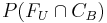, which is the probability of undesirable flow rates and valve configuration B.

If we use Apparatus A 79% of the time then the probability of using Apparatus B would be 21% or :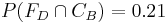, which is the probability of using configuration B and getting desired flow rates.

Additionally, we are given :, which is the probability of a desired flow rate, given that configuration B is used.

Then, which is the probability that an undesirable flow rate is obtained, given that configuration B is used.

The information from above is then used below:, which is the probability of getting an undesirable flow rate and configuration B which is found by taking the probability of getting an undesirable flow rate given that configuration B is used and multiplying that by the probability of a desirable flow rate and configuration B.

Example $$\PageIndex{1}$$

Consider the scenario in which you toss a fair die 12 times. What is the probability that each face value (1-6) will occur exactly twice?

Solution

The probability can be determined using a multinomial distribution in which 6 outcomes are possible. The individual probabilities are all equal given that it is a fair die, p = 1/6. The total number of trials N is 12, and the individual number of occurrences in each category n is 2.

$P(2,2,2,2,2,2)-\frac{12 !}{2 ! 2 ! 2 ! 2 ! 2 ! 2 !} *\left(\frac{1}{6}\right)^{2}\left(\frac{1}{6}\right)^{2}\left(\frac{1}{6}\right)^{2}\left(\frac{1}{6}\right)^{2}\left(\frac{1}{6}\right)^{2}\left(\frac{1}{6}\right)^{2}-0.003488 \nonumber$

Therefore, the probability of rolling exactly 2 of each face value on a fair die is about 0.35%.

Example $$\PageIndex{2}$$

A bowl has 2 maize marbles, 3 blue marbles and 5 white marbles. A marble is randomly selected and then placed back in the bowl. You do this 5 times. What is the probability of choosing 1 maize marble, 1 blue marble and 3 white marbles?

Solution

• N is the number of trials = 5
• k is the number of possible outcomes = 3
• ni is the number of occurrences of outcome i
• pi is the probability of seeing outcome i

The three possible outcomes are choosing a maize marble, a blue marble or a white marble.

We must determine ni and pi to solve the multinomial distribution.

The number of occurrences of the outcome are the number of times we wish to see each outcome. These are given in the problem statement.

nmaize = 1

nblue = 1

nwhite = 3

The probability of seeing each outcome is easy to find. For example, there are two maize marbles in the bowl of 10, so the probability of choosing a maize marble is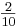.

pmaize =pblue =pwhite =We can now solve the multinomial distribution as shown below. The probability of choosing 1 maize marble, 1 blue marble and 3 white marbles is 0.15.

$P(1,1,3)=\frac{5 !}{1 ! 1 ! 3 !} *\left(\frac{2}{10}\right)^{1}\left(\frac{3}{10}\right)^{1}\left(\frac{5}{10}\right)^{3}=0.15$

Example $$\PageIndex{3}$$

Two valves are used to control the flow of liquid out of a storage tank (Tank 1) in to another storage tank (Tank 2) and controlled by Valves 1 and 2 as seen below. The valves exist in two states: open and closed.The following table describes the four different valve configurations and the frequency of the desired flow for each valve configuration based on experimental data.

Configuration

Valve 1

Valve 2

Probability of that configuration having the best flow

1

Open

Closed

0.305

2

Open

Open

0.476

3

Closed

Open

0.218

4

Closed

Closed

0.001

To reduce costs, two different apparatuses with a similar configuration, but with different pipe dimensions are being considered to replace the original apparatus. Below are data tables for each potential replacement apparatus with different valve configurations and the number of times out of 100 measurements that the valve configuration gave the desired flow.

Apparatus 1

Configuration

Valve 1

Valve 2

# of Best
Flow

1

Open

Closed

42

2

Open

Open

37

3

Closed

Open

21

4

Closed

Closed

0

Apparatus 2

Configuration

Valve 1

Valve 2

# of Best
Flow

1

Open

Closed

20

2

Open

Open

22

3

Closed

Open

56

4

Closed

Closed

2

Based on the information above, which apparatus is more like the desired model? By how much?

Solution

With the 4 different valve configurations, multinomial distribution can be utilized to calculate the probability of a measurement. The goal here is to find the model with the highest probability density function, because that one is more like the desired model.

The probability of a measurement can be calculated as follows given that there are 4 different possible valve configurations (see section on pdf for more information on where this equation came from):

$P\left(n_{1}, n_{2}, n_{3}, n_{4}\right)=\frac{N !}{n_{1} ! n_{2} ! n_{3} ! n_{4} !} p_{1}^{n_{1}} p_{2}^{n 2} p_{3}^{n 3} p_{4}^{n 4} \nonumber$

where

• ni = total number of measurements with the best flow rate from each valve configuration
• i = configuration
• pi = probability of that configuration having the best flow
• N = Total # of observations

The calculation for the probability of each apparatus can be done similarly:

Apparatus 1:Apparatus 2: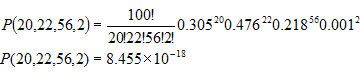Based on the calculations above for both apparatuses, Apparatus 1 is more like the desired model. Apparatus 1 has a higher probability density function, based on the relative likelihood of each configuration flow.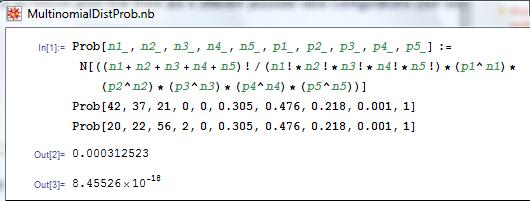This figure shows Mathematica code that can be used in order to solve the probability of a multinomial distribution. The n values are the number of occurrences of each outcome and the p values are the probabilities of eachout come. The function is set for a multinomial distribution with five different outcomes. However, it can be used for multinomial distributions with fewer outcomes by setting the unused n values to 0 and the unused p values to any number other than 0. This figure also shows the probabilities calculated from Apparatus 1 and Apparatus 2.

Example $$\PageIndex{4}$$

A runaway reaction occurs when the heat generation from an exothermic reaction exceeds the heat loss. Elevated temperature increases reaction rate, further increasing heat generation and pressure buildup inside the reactor. Together, the uncontrolled escalation of temperature and pressure inside a reactor may cause an explosion.

The precursors to a runaway reaction - high temperature and pressure - can be detected by the installation of reliable temperature and pressure sensors inside the reactor. Runaway reactions can be prevented by lowering the temperature and/or pressure inside the reactor before they reach dangerous levels. This task can be accomplished by sending a cold inert stream into the reactor or venting the reactor.

Les Lloyd is a process engineer at the Miles Reactor Company that has been assigned to work on a new reaction process. Using historical data from all the similar reactions that have been run before, Les has estimated the probabilities of each outcome occurring during the new process. The potential outcomes of the process include all permutations of the possible reaction temperatures (low and high) and pressures (low and high). He has combined this information into the table below:

Outcome

Temperature

Pressure

Probability

1

high

high

0.013

2

high

low

0.267

3

low

high

0.031

4

low

low

0.689

Worried about risk of runaway reactions, the Miles Reactor Company is implementing a new program to assess the safety of their reaction processes. The program consists of running each reaction process 100 times over the next year and recording the reactor conditions during the process every time. In order for the process to be considered safe, the process outcomes must be within the following limits:

Outcome

Temperature

Pressure

Frequency

1

high

high

n1 = 0

2

high

low3

low

high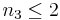4

low

low

n4 = 100 − n1n2n3

Help Les predict whether or not the new process is safe by answering the following question: What is the probability that the new process will meet the specifications of the new safety program?

Solution

The probability of the safety guidelines being met is given by the following CDF expression: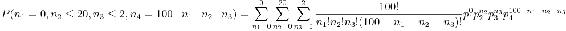where

• N is the number of trials
• k is the number of possible outcomes
• ni is the number of occurrence of outcome i
• pi is the probability of seeing outcome i
• ci is the maximum number of occurrence of outcome i

This CDF expression can be evaluated using the following commands in Mathematica:

<< Needs["MultivariateStatistics`"]
<< multinomial = MultinomialDistribution[100, {0.013, 0.267, 0.031, 0.689}]
<< CDF[multinomial, {0, 20, 2, 78}]

The result is:

$P\left(n_{-}=0, n_{2} \leq 20, n_{3} \leq 2, n_{4}=1000-n_{1}-n_{2}-n_{3}\right)=0.00132705$

Based on this probability calculation, it appears unlikely that this new process will pass the new safety guidelines.